Courses

# Test: Problems on Free Body-1

## 10 Questions MCQ Test Physics Class 11 | Test: Problems on Free Body-1

Description
This mock test of Test: Problems on Free Body-1 for JEE helps you for every JEE entrance exam. This contains 10 Multiple Choice Questions for JEE Test: Problems on Free Body-1 (mcq) to study with solutions a complete question bank. The solved questions answers in this Test: Problems on Free Body-1 quiz give you a good mix of easy questions and tough questions. JEE students definitely take this Test: Problems on Free Body-1 exercise for a better result in the exam. You can find other Test: Problems on Free Body-1 extra questions, long questions & short questions for JEE on EduRev as well by searching above.
QUESTION: 1

### Force that produces an acceleration of 1 ms−2 in a body of mass of 1 kg is called

Solution:

We know that F = ma, and for m = 1kg and a = 1m/s2
We get F = 1N which is one newton

QUESTION: 2

### A horizontal force of 100 N pulls two masses 5 kg and 10 kg tied to each other by a light string. What is the tension in the string if the force is applied on 10 kg mass?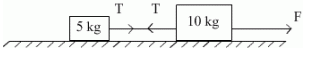Solution:

At first considering both blocks as one system with only one external force F
We get common acceleration at right be a = 100/15 m/s2
Now considering 10 kg block
We get F - T = 10a
i.e. T = 100  - 10(100/15)
= 100 (1 - 2/3)
= 33.33 N

QUESTION: 3

### A man weighing 100 kgf carries a load of 10 kgf on his head. He jumps from tower with that load. What will be the weight of load experienced by the man.

Solution:
QUESTION: 4

For a body to be able to loop a vertical circle of radius R, the minimum velocity required at its lowest point is:

Solution:

For a body able to loop a vertical circle, the tension in the string must not be lesser than zero at the highest point. Hence when the tension at the highest point is zero we will obtain the minimum velocity. I.e.
At the highest point as T = 0, hence we get
Mg = Mv2/R
(comparing the centripetal acceleration with weight, where R is radius of vertical circle)
Hence we get the minimum velocity at the topmost point to be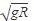Thus if we apply the work energy theorem upon the particle from its lowermost point to its top most point, we get net displacement as 2R and thus minimum velocity at lowermost point to be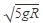QUESTION: 5

A block of 5 kg mass rests on a horizontal floor. The action of the block on the floor is

Solution:

Weight of the block, mg = 5kg x 10 m/s2 = 50 N.
According to Newton’s third law, the action of the block, that is the force exerted on the floor by the block is equal to 50 N in magnitude and is directly vertically downward.

QUESTION: 6

A lift is moving down with the acceleration 3 m/s2. A ball is released 1.7 m above the the lift floor. How long will it take to hit the lift floor.

Solution:

As the lift itself is accelerating hence the net downwards acceleration of the ball will be 9.8 - 3
= 6.8 m/s2
Thus by applying second equation of motion we get, t =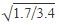= 0.71

QUESTION: 7

A body of mass 2 kg is hung on a spring balance mounted vertically in a lift. If the lift moves up with an acceleration equal to the acceleration due to gravity, the reading on the spring balance will be

Solution:

As the lift moves upwards but the spring feels itself at rest hence we need to compensate the non inertial frame by adding an appropriate pseudo force to treat it as an inertial frame. Hence the pseudo force to be applied acts on every mass in the lift which is equal to mass x acceleration (=g) downwards.
Hence the tension in the spring would be 40N (20 due to weight and 20 pseudo). Thus the reading would be 4kg.

QUESTION: 8

A block of mass m is pulled by applying a force F at an angle θ with the horizontal surface. Its acceleration will be given by

Solution:

When the force F is applied at some angle, only the component of it which is parallel to the surface would provide acceleration to it. The other one would be balanced by the normal force. Hence as the component parallel to the surface is F.cosΘ , we get a = F.cosΘ / m

QUESTION: 9

Consider two blocks of masses m1 and m2 placed in contact with each other on a friction less horizontal surface. Let a force F be applied on block of mass m1, then the value of contact force between the blocks is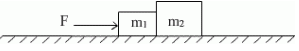Solution:

At first consider both blocks as one system then we get the common acceleration of the system,  a =  F / m1 + m2
Now if we see only mass m1
We get that for contact force f,
F - f = m1a
Thus we get f = F - m1 F / m1 + m2
=  m2F /  m1 + m2

QUESTION: 10

The couple and the other two force systems in free body diagrams can be easily simplified.

Solution:

Both of them are vector quantities. And both of them can be easily simplified. If taken in the vector form then the task is even easier. Thus it is not necessary for the force or the couple to be vector only, even if the magnitude is taken, the simplification is done in the 2D.